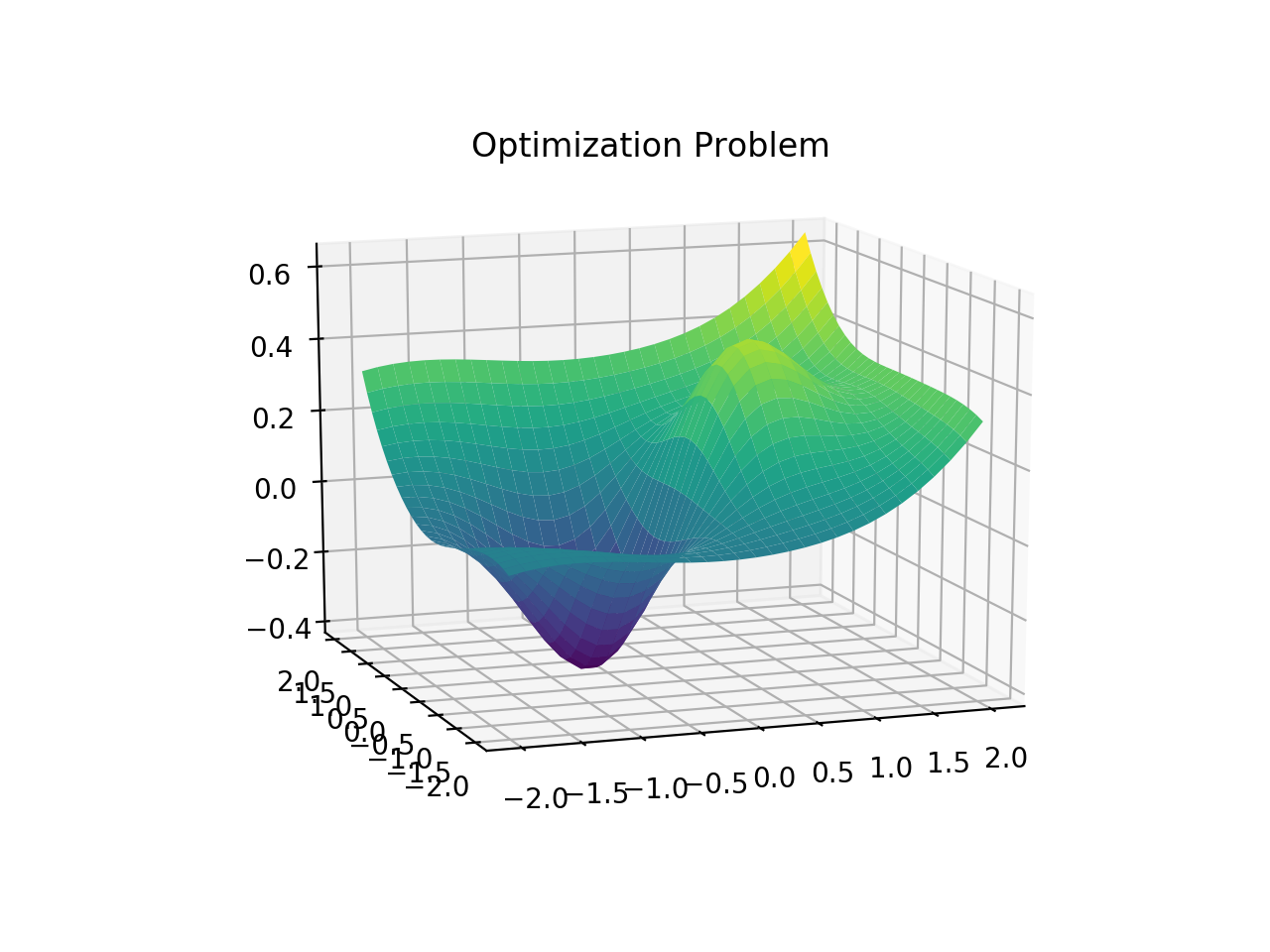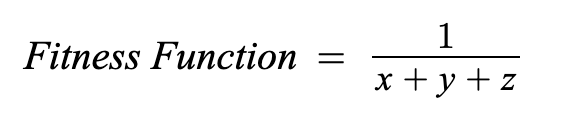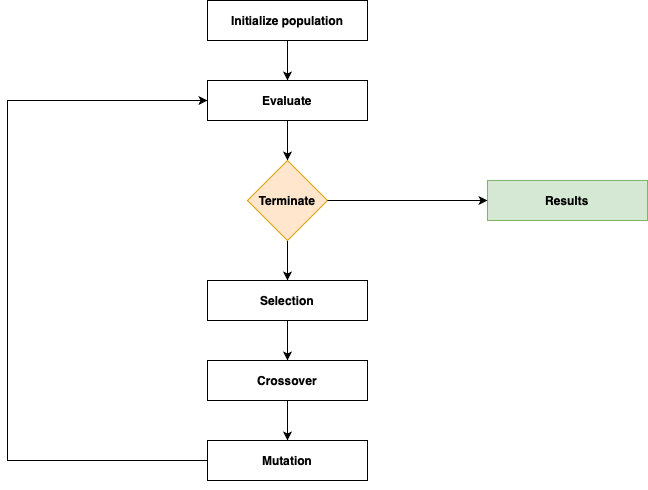1626429780

# Introduction to Genetic Algorithm

## What is Optimization?

• Making something better.
• Increase efficiency.

## Optimization problem

• A problem in which we have to find the values of inputs (also called solutions or decision variables) from all possible inputs in such a way that we get the “best” output values.
• Definition of “best”- Finding the values of inputs that result in a maximum or minimum of a function called the objective function.
• There can be multiple objective functions as well (depends on the problem).

## Optimization algorithm

An algorithm used to solve an optimization problem is called an optimization algorithm.

## Evolutionary Algorithms

Algorithms that simulate physical and/or biological behavior in nature to solve optimization problems.

## Genetic Algorithm (GA)

• It is a subset of evolutionary algorithms that simulates/models Genetics and Evolution (biological behavior) to optimize a highly complex function.
• A highly complex function can be:
• 1. Very difficult to model mathematically.
• 2. Computationally expensive to solve. Eg. NP-hard problems.
• 3. Involves a large number of parameters.

## Background of GA

• Introduced by Prof. John Holland in 1965.
• The first article on GA was published in 1975.
• GA is based on two fundamental biological processes:
• 1. Genetics (by G.J. Mendel in 1865): It is the branch of biology that deals with the study of genes, gene variation, and heredity.
• 2. Evolution (by C. Darwin in 1875): It is the process by which the population of organisms changes over generations.

## Natural selection in Evolution

1. A population of individuals exists in an environment with limited resources.
2. Competition for those resources causes the selection of those fitter individuals that are better adapted to the environment.
3. These individuals act as seeds for the generation of new individuals through recombination and mutation.
4. Evolved new individuals act as initial population and Steps 1 to 3 are repeated.

## Applications of GA

• 1. Acoustics
• 2. Aerospace Engineering
• 3. Financial Markets
• 4. Geophysics
• 5. Materials Engineering
• 6. Routing and Scheduling
• 7. Systems Engineering

## Problems with GA

• 1. Population Selection Problem
• 2. Defining Fitness Function
• 3. Premature or rapid convergence of GA
• 4. Convergence to Local Optima

#evolutionary-algorithms #data-science #genetic-algorithm #algorithm

## Buddha Community1626429780

## What is Optimization?

• Making something better.
• Increase efficiency.

## Optimization problem

• A problem in which we have to find the values of inputs (also called solutions or decision variables) from all possible inputs in such a way that we get the “best” output values.
• Definition of “best”- Finding the values of inputs that result in a maximum or minimum of a function called the objective function.
• There can be multiple objective functions as well (depends on the problem).

## Optimization algorithm

An algorithm used to solve an optimization problem is called an optimization algorithm.

## Evolutionary Algorithms

Algorithms that simulate physical and/or biological behavior in nature to solve optimization problems.

## Genetic Algorithm (GA)

• It is a subset of evolutionary algorithms that simulates/models Genetics and Evolution (biological behavior) to optimize a highly complex function.
• A highly complex function can be:
• 1. Very difficult to model mathematically.
• 2. Computationally expensive to solve. Eg. NP-hard problems.
• 3. Involves a large number of parameters.

## Background of GA

• Introduced by Prof. John Holland in 1965.
• The first article on GA was published in 1975.
• GA is based on two fundamental biological processes:
• 1. Genetics (by G.J. Mendel in 1865): It is the branch of biology that deals with the study of genes, gene variation, and heredity.
• 2. Evolution (by C. Darwin in 1875): It is the process by which the population of organisms changes over generations.

## Natural selection in Evolution

1. A population of individuals exists in an environment with limited resources.
2. Competition for those resources causes the selection of those fitter individuals that are better adapted to the environment.
3. These individuals act as seeds for the generation of new individuals through recombination and mutation.
4. Evolved new individuals act as initial population and Steps 1 to 3 are repeated.

## Applications of GA

• 1. Acoustics
• 2. Aerospace Engineering
• 3. Financial Markets
• 4. Geophysics
• 5. Materials Engineering
• 6. Routing and Scheduling
• 7. Systems Engineering

## Problems with GA

• 1. Population Selection Problem
• 2. Defining Fitness Function
• 3. Premature or rapid convergence of GA
• 4. Convergence to Local Optima

#evolutionary-algorithms #data-science #genetic-algorithm #algorithm1593350760

## Genetic Algorithm (GA): A Simple and Intuitive Guide

Learn what are metaheuristics and why we use them sometimes instead of traditional optimization algorithms. Learn the metaheuristic Genetic Algorithm (GA) and how it works through a simple step by step guide.

#genetic-algorithm #algorithms #optimization #metaheuristics #data-science #algorithms1624882500

## A Guide to Genetic ‘Learning’ Algorithms for Optimization

In a broader mathematical or computational perspective, an optimization problem is defined as a problem of finding the best solution from all feasible solutions. In terms of Machine Learning and Artificial Intelligence, two significant algorithms that perform these tasks are Reinforcement Learning and Genetic Algorithms. They serve the purpose of finding the ‘best fit’ solutions from a range of possible solutions for a given problem statement. In the article that follows below, we will be working closely on these algorithms and will see their implementation in action on an Image Processing problem.

### What is a Genetic Algorithm (GA)?

Genetic algorithms are random, adaptive heuristic search algorithms that act on a population of doable solutions. they need loosely supported the mechanics of population biology and choice.

Genetic algorithms are based on the ideas of natural selection and genetics. New solutions are typically made by ‘mutating’ members of this population, and by ‘mating’ 2 resolutions along to create a replacement solution.

The upper solutions are selected to breed and change and so the more severe ones are discarded. They are probabilistic search methods; this implies that the states that they explore are not determined entirely by the properties of the problems. A random method helps to guide the search. Genetic algorithms are utilized in AI like different search algorithms utilized in AI — to seem for potential solutions to hunt out one that solves the matter.

#artificial-intelligence #genetics #algorithms #data-science #genetic ‘learning’ algorithms1598775060

## Optimization Techniques: Genetic Algorithm

In complex machine learning models, the performance usually depends on multiple input parameters. In order to get the optimal model, the parameters must be properly tuned. However, when there are multiple parameter variables, each ranging across a wide spectrum of values, there are too many possible configurations for each set of parameters to be tested. In these cases, optimization methods should be used to attain the optimal input parameters without spending vast amounts of time finding them.In the diagram above, it shows the distribution of the model based on only two parameters. As evident in the example shown, it is not always an easy task to find the maximum or minimum of the curve. This is why optimization methods and algorithms are crucial in the field of machine learning.

## Genetic Algorithm

The most commonly used optimization strategy are Genetic Algorithms. Genetic Algorithms are based off of Darwin’s theory of natural selection. It is relatively easy to implement and there is a lot of flexibility for the setup of the algorithm so that it can be applied to a wide range of problems.

### Choosing a Fitness Function

To start off, there must be a fitness function that measures how well a set of input parameters perform. Solutions with a higher fitness derived from a fitness function will be better than ones with a lower fitness.

For example, if a solution has a cost of x + y + z, then the fitness function should try to minimize the cost. This can be done with the following fitness function#genetic-algorithm #optimization #genetics #optimization-algorithms #machine-learning1595543700

## An Introduction to Genetic Algorithms

Genetic Algorithms (GAs) are a part of Evolutionary Computing (EC), which is a rapidly growing area of Artificial Intelligence (AI). It inspired by the process of biological evolution based on Charles Darwin’s theory of natural selection, where fitter individuals are more likely to pass on their genes to the next generation. We, as human beings, also are the result of thousands of years of evolution.

# History of Genetic Algorithms

The GA, developed by John Holland and his collaborators in the 1960s and 1970s.

• As early as 1962, John Holland’s work on adaptive systems¹ laid the foundation for later developments.
• By the 1975, the publication of the book “Adaptation in Natural and Artificial Systems”², by Holland and his students and colleagues.

The GA got popular in the late 1980s by was being applied to a broad range of subjects that are not easy to solve using other techniques.

In 1992, John Koza has used genetic algorithm to evolve programs to perform certain tasks. He called his method “genetic programming” (GP)³.

# What is evolution in the real world?

For thousands of years, humans have acted as agents of genetic selection, by breeding offspring with desired traits. All our domesticated animals and food crops are the results. Let review the genetic terms in nature as follows.

• Each cell of a living thing contains chromosomes — strings of DNA.
• Each chromosome contains a set of genes — blocks of DNA
• Each gene determines some aspect of the organism (like eye colour)
• A collection of genes is sometimes called a genotype
• A collection of aspects (like eye colour) is sometimes called a phenotype
• Reproduction (crossover) involves recombination of genes from parents and then small amounts of mutation (errors) in copying
• The fitness of an organism is how much it can reproduce before it dies
• Evolution based on “survival of the fittest”

# What’s Genetic Algorithm in Computer Science?

Genetic Algorithms are categorized as global search heuristics. A genetic algorithm is a search technique used in computing to find true or approximate solutions to optimization and search problems. It uses techniques inspired by biological evolution such as inheritance, mutation, selection, and crossover.five steps of a genetic algorithm

We look at the basic process behind a genetic algorithm as follows.

Initialize population: genetic algorithms begin by initializing a Population of candidate solutions. This is typically done randomly to provide even coverage of the entire search space. A candidate solution is a Chromosome that is characterized by a set of parameters known as Genes.

**Evaluate: **next, the population is evaluated by assigning a fitness value to each individual in the population. In this stage we would often want to take note of the current fittest solution, and the average fitness of the population.

#python #genetic-algorithm #technology #algorithms